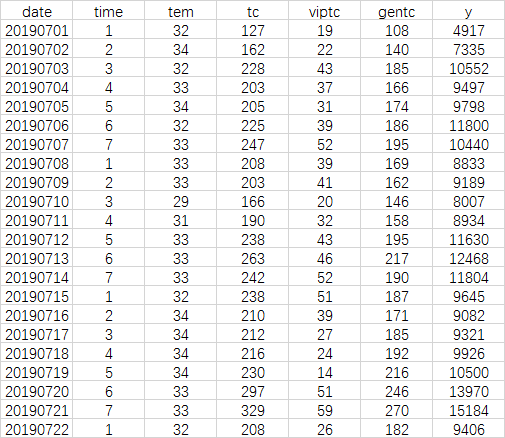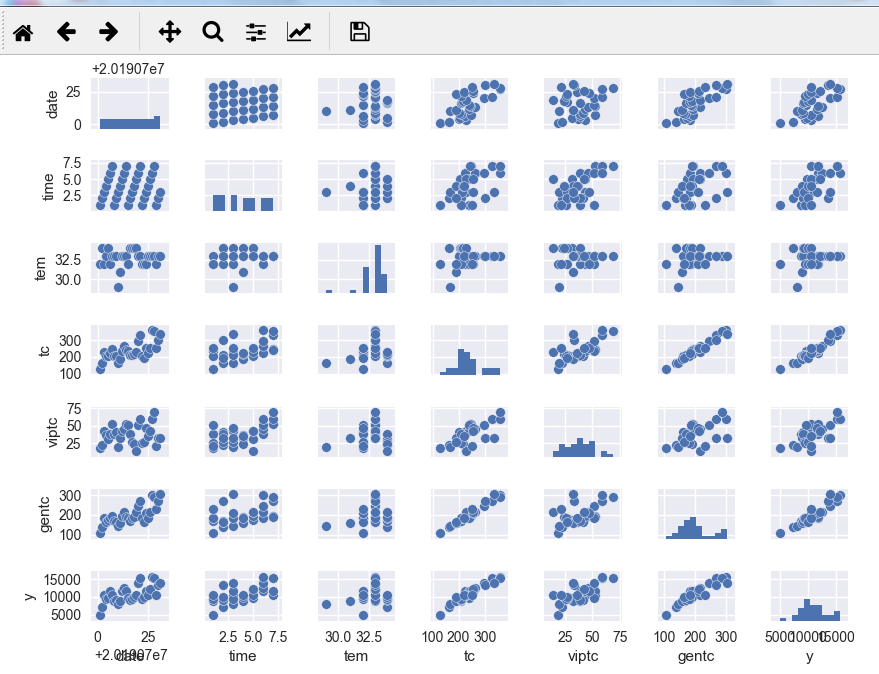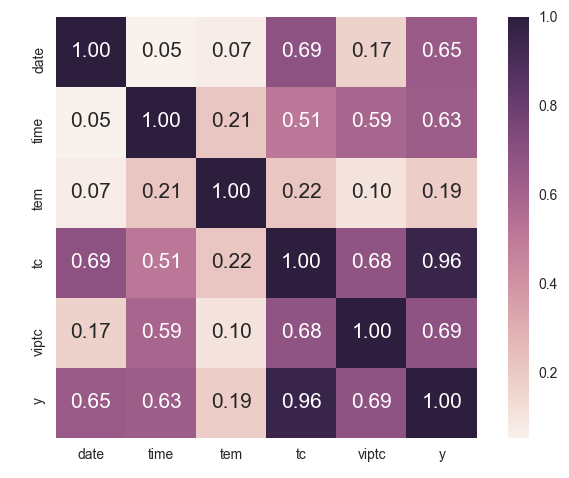# 线性回归分析实例（餐饮数据）python皮尔逊相关性学习笔记 同时被 3 个专栏收录3 篇文章 0 订阅

## 实例

date：日期 time：星期几 tem：历史温度 y：营业额 tc：总单数 viptc：会员单数import pandas as pd
import numpy as np
import matplotlib.pyplot as plt
import seaborn as sns
from sklearn.linear_model import LinearRegression


data_df=pd.read_csv(a)
sns.pairplot(data_df,size=1)
plt.show()seaborn计算绘制皮尔逊相关性系数并汇图

cols=['date','time','tem','tc','viptc','y']
cm =np.corrcoef(data_df[cols].values.T)
hm = sns.heatmap(cm,cbar=True,annot=True,square=True,fmt=".2f",
annot_kws={"size":15},yticklabels=cols,xticklabels=cols)
plt.show()cols2=[['date','time','tc','viptc','y']]
mo=LinearRegression()
x=data_df[['date','time','tc','viptc']]
y=data_df['y']#实际营业额
mo.fit(x,y)
pre_y=mo.predict(x)#预测营业额


## 结论

11-2818102-025220
08-27
01-28
03-254434
05-107
02-03371
11-24
04-28
04-258万+
08-22
05-028万+
02-041951
04-201140
11-281
10-241708点击重新获取扫码支付余额充值